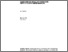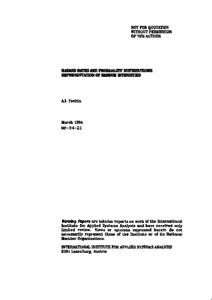# Hazard Rates and Probability Distributions: Representation of Random Intensities

Yashin, A.I. (1984). Hazard Rates and Probability Distributions: Representation of Random Intensities. IIASA Working Paper. IIASA, Laxenburg, Austria: WP-84-021Preview Text WP-84-021.pdf Download (1MB) | Preview

## Abstract

Recent attempts to apply the results of martingale theory in probability theory have shown that it is first necessary to interpret this abstract mathematical theory in more conventional terms. One example of this is the need to obtain a representation of the dual predictable projections (compensators) used in martingale theory in terms of probability distributions. However, up to now a representation of this type has been derived only for one special case.

In this paper, the author gives probabilistic representations of the dual predictable projection of integer-valued random measures that correspond to jumps in a semimartingale with respect to the sigma-algebras generated by this process. The results are of practical importance because such dual predictable projections are usually interpreted as random intensities or hazard rates related to jumps in trajectories: applications are found in such fields as mathematical demography and risk analysis.

Item Type: Monograph (IIASA Working Paper) System and Decision Sciences - Core (SDS) IIASA Import 15 Jan 2016 01:55 27 Aug 2021 17:12 https://pure.iiasa.ac.at/2497View Item